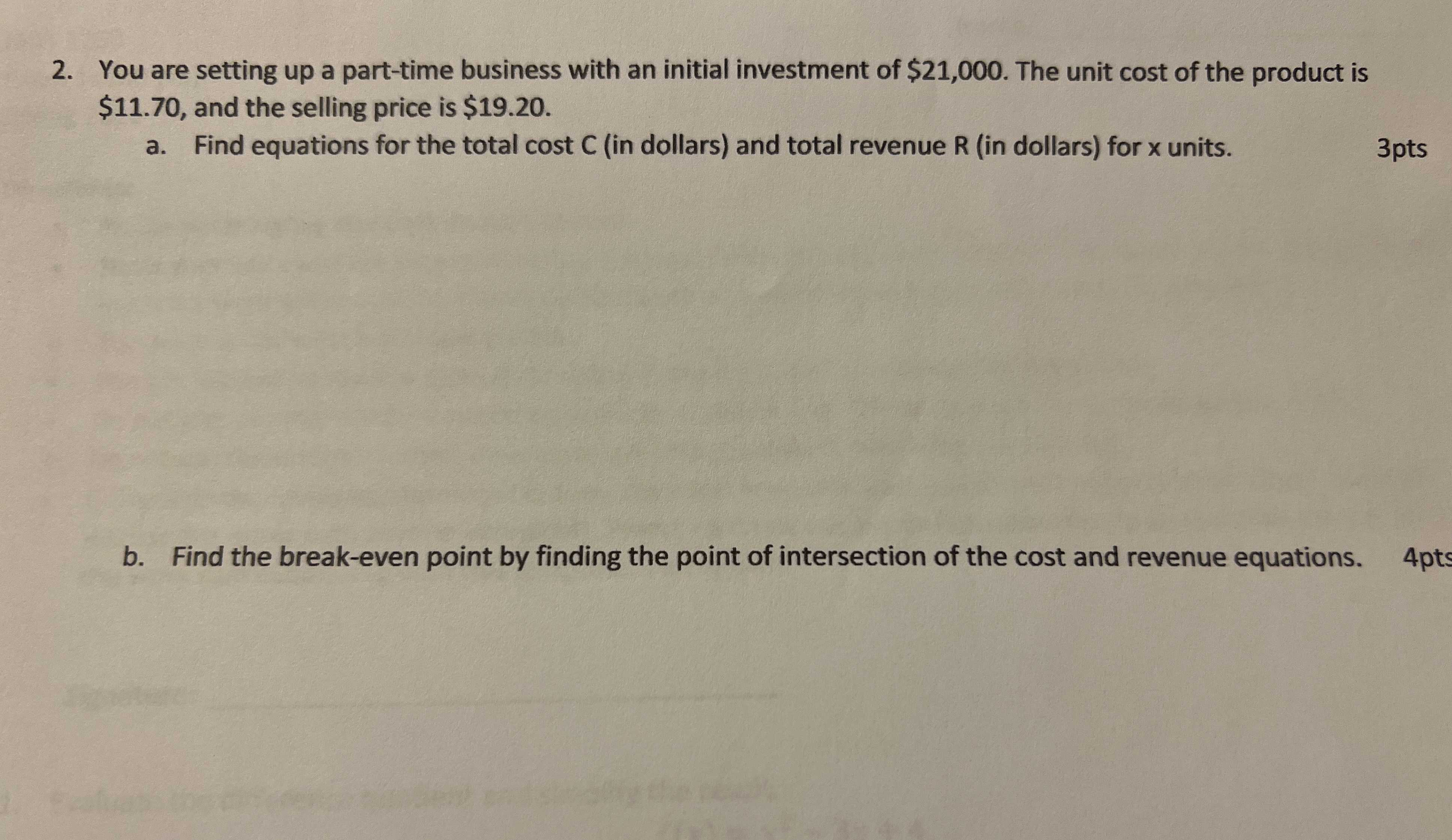### ¿Todavía tienes preguntas de matemáticas?

Pregunte a nuestros tutores expertos
Algebra
Pregunta2. You are setting up a part-time business with an initial investment of $$\ 21,000$$ . The unit cost of the product is $$\ 11.70$$ , and the selling price is $$\ 19.20$$ . a. Find equations for the total cost $$C$$ (in dollars) and total revenue $$R$$ (in dollars) for $$x$$ units. b. Find the break-even point by finding the point of intersection of the cost and revenue equations.